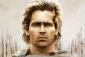# Calculate $0.5 + 0.5^2 + 0.5^3 + \ldots + 0.5^{20}$

8 posts / 0 new
Jhun VertCalculate $0.5 + 0.5^2 + 0.5^3 + \ldots + 0.5^{20}$

Using your calculator, find the value of
$0.5 + 0.5^2 + 0.5^3 + \, ... \, + 0.5^{20}$

esmilitarUSING Mode 3,5 e^x
input the following data
X Y
1 0.5
2 0.52
3 0.53
Press AC
Shift Sigma (AlphaX shift stat y caret, 1,20)=0.9999990463 The sum of 1st to the 20th term of the sequence

AlexanderMode 1:esmilitarThank you for this the best shortcut method..

AlexanderWelcome

BaileyKicksAssHey! btw do you know the bartender problem that is a bit similar to your task? Here it is: an infinitive quantity of mathematicians walks in the bar. First mathematician orders a glass of beer, the second one orders a half of glass of beer, the third one orders a 1/4 of glass of beer, the next one orders a 1/8 of glass of beer....and so on. The issue is how many FULL glasses of beer should bartender give to them? I work at https://handmadewritings.com as a content writer, but sometimes I like to solve math problems! waiting for your responces.

KMST

Suppose the bartender had only enough beer for 2 glasses.
After the first order, the bartender thinks "I have only enough beer left for 1 glass."
After the second order, the bartender thinks "I have only enough beer left for 1/2 glass."
After the third order, the bartender thinks "I have only enough beer left for 1/4 glass."
After the fourth order, the bartender thinks "I have only enough beer left for 1/8 glass."
As long as only those crazy mathematicians show up, the bartender would theoretically never run out of liquid to fill the orders. The world would run out of mathematicians before there is only a single molecule left. However, at some point less than a drop would have to be served, and that would pose a technical problem. Also, the flavor would start changing as the liquid left became depleted of minor flavor components.

james (guest)

• Mathematics inside the configured delimiters is rendered by MathJax. The default math delimiters are $$...$$ and $...$ for displayed mathematics, and $...$ and $...$ for in-line mathematics.